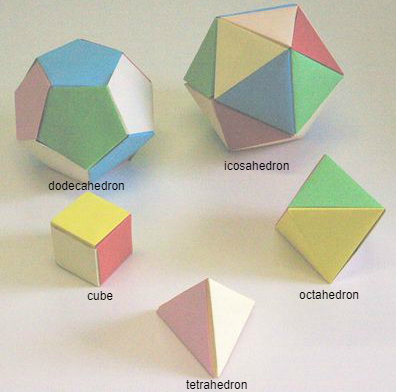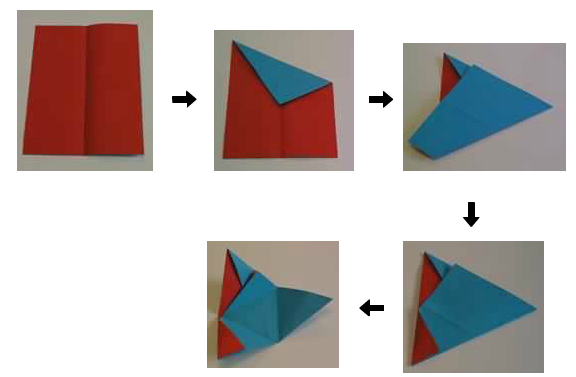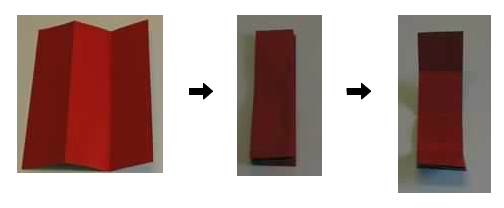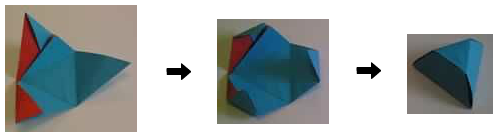# Paper Folding - Models of the Platonic Solids

##### Age 11 to 16

Published 2007 Revised 2012

This article describes how to build models of the Platonic solids using sheets of paper.

Suppose you have a collection of regular polygons and each polygon has the same number of faces. For example, the polygons may all be regular squares. Connect the polygons together edge to edge to form a structure like a shell which completely encloses a region. For example, six regular squares can be connected together to form a cube. Such a structure is known as a polyhedron . The polyhedron is regular if, informally speaking, it has as much symmetry as possible. To appreciate better what regular means, position the polyhedron in front of you so that you are directly facing a vertex, and take a photograph. Adjust the polyhedron so that you face a different vertex and take another photograph. The polyhedron is regular if, after rotating the photographs suitably, the images in the two photographs appear identical, no matter the choice of vertices.

There are exactly five such regular polyhedra (shown below), and they are known as the Platonic solids .Here are some simple recipes for building models of the Platonic solids out of paper. I use A4 paper - that is rectangular paper such that the length of the long side of the rectangle divided by the length of the short side is equal to the square-root of 2. The dodecahedron and icosahedron models have appeared previously on the NRICH web-site.

For the purposes of illustration, the pieces of A4 paper used below are coloured red on one side and blue on the other.

The tetrahedron.

Fold an A4 sheet of paper as shown below. The result is a tetrahedron net, but it will not maintain the shape of a tetrahedron of its own accord. Make another identical net, then slot the two nets together to form a rigid tetrahedron.The octahedron.

Make four tetrahedron nets following the recipe above for each one. Slot them together to form an octahedron . (To do this, slot two nets together to form what looks like a square pyramid with flaps attached. Do the same for the other two nets. Now slot these two square pyramid objects together.)

The cube.

This model only truly represents a cube if the ratio of the short to long sides of your rectangular piece of paper is 3:4. You can achieve this ratio by removing a 17mm width strip from a sheet of A4 paper to decrease the length of the longer edge of the A4 paper. Repeat the procedure shown below for three separate sheets of paper, and interlock the resulting three objects to form a cube.The dodecahedron.

The object that results from this recipe is a nearly regular pentagon with two flaps. Make twelve of these objects and join them together by the flaps to form a dodecahedron.

The icosahedron.

We continue from the final stage of the tetrahedron recipe to produce a truncated tetrahedron, shown below. Make twenty of these truncated tetrahedra and glue them together to form an icosahedron .These models were developed in collaboration with Simon Joyce in the National University of Ireland, Maynooth. Excessively dark photographs taken by Kurt Falk.